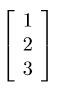# Find a 3x3 Matrix such that....

## Homework Statement

Find a non zero matrix(3x3) that does not havein its range. Make sure your matrix does as it should.

## The Attempt at a Solution

[/B]
I know a range is a set of output vectors, Can anyone help me clarify the question?
I'm just not sure specifically what its asking of me, in regards to the matrix Im looking for.

HallsofIvy
Homework Helper
You are asked to find a 3 by 3 matrix, A, such that Ax is NOT equal to $\begin{bmatrix} 1 \\ 2 \\ 3 \end{bmatrix}$ for any x. This does not have a single correct aswer. The trivial solution, the zero matrix, would be such a matrix because the zero matrix times any x is the 0 vector- but that is specifically excluded- you must give a "non-zero" matrix. There are still an infinite number of such matrices. What about a matrix that makes the first component of any vector 0? Or the second or third component? (But not all three).

Ray Vickson
Homework Helper
Dearly Missed

## Homework Statement

Find a non zero matrix(3x3) that does not havein its range. Make sure your matrix does as it should.

## The Attempt at a Solution

[/B]
I know a range is a set of output vectors, Can anyone help me clarify the question?
I'm just not sure specifically what its asking of me, in regards to the matrix Im looking for.

You are being asked to find a ##3 \times 3## matrix ##A## for which the vector ##\vec{r} = \langle 1,2,3 \rangle^T ## (transpose) is an impossible output; that is, you want##A \vec{x} \neq \vec{r}## for ALL input vectors ##\vec{x} \in R^3##.

We haven't learned about tranpose yet in class(but i just briefly looked it up right now)

What is the purpose of taking the transpose of the the column vector 1 2 3 in this case?

So I find this A= 3x3 matrix that when I multiply it by any column vector(x,y,z) the ouput does not equal r<1,2,3>T is this correct?

Ray Vickson
Homework Helper
Dearly Missed
We haven't learned about tranpose yet in class(but i just briefly looked it up right now)

What is the purpose of taking the transpose of the the column vector 1 2 3 in this case?

So I find this A= 3x3 matrix that when I multiply it by any column vector(x,y,z) the ouput does not equal r<1,2,3>T is this correct?

Is that not what I just said?

Anyway, writing a transpose simplifies typing. Instead of taking 3 lines to write the column vector like this:
$$\left[ \begin{array}{c}1\\2\\3 \end{array} \right]$$
we can write it in one line, like this: ##(1,2,3)^T## or ##\langle 1,2,3 \rangle^T##, as the transpose of a row-vector.

Last edited:
WWGD
Gold Member
You can use rank-nullity: just use a matrix that "kills" the vector ## (1,2,3)^T ##, say.

Is that not what I just said?

Anyway, writing a transpose simplifies typing. Instead of taking 3 lines to write the column vector like this:
$$\left[ \begin{array}{c}1\\2\\3 \end{array} \right]$$
we can write it in one line, like this: ##(1,2,3)^T## or ##\langle 1,2,3 \rangle^T##, as the transpose of a row-vector.

Thanks for explaining that to me.

You can use rank-nullity: just use a matrix that "kills" the vector ## (1,2,3)^T ##, say.

Sounds interesting. Unfortunately we have not covered this yet(2nd week in) but I am watching a youtube videos to see how this "Killing" things works.

You are asked to find a 3 by 3 matrix, A, such that Ax is NOT equal to $\begin{bmatrix} 1 \\ 2 \\ 3 \end{bmatrix}$ for any x. This does not have a single correct aswer. The trivial solution, the zero matrix, would be such a matrix because the zero matrix times any x is the 0 vector- but that is specifically excluded- you must give a "non-zero" matrix. There are still an infinite number of such matrices. What about a matrix that makes the first component of any vector 0? Or the second or third component? (But not all three).

thanks for your input. I had a feeling thats exactly what it meant, but sometimes i doubt my self with these kind of things. and when I was trying to understand the problem I figured there was more than one answer(you confirmed it)

When you refer to x, does that mean the column vector with x,y,z (any x ,y,z, values) which when multiplied by Matrix A does not equal specified range?

Ray Vickson
Homework Helper
Dearly Missed
Sounds interesting. Unfortunately we have not covered this yet(2nd week in) but I am watching a youtube videos to see how this "Killing" things works.

I don't know if you have had the following material yet, but in 2d or 3d it is "obvious" from diagrams: given a fixed vector ##\vec{u}##, any vector ##\vec{v}## can be decomposed into a component ##\vec{v}_{||}## parallel to ##\vec{u}## and a component ##\vec{v}_{\perp}## perpendicular to ##\vec{u}##. (Think of decomposing a force in Physics into components parallel and perpendicular to some given direction.)

What would happen if, for every vector ##\vec{v}## you took the perpendicular component ##\vec{v}_{\perp}##? Is the operation ##\vec{v} \rightarrow \vec{v}_{\perp}## a linear operation?

WWGD